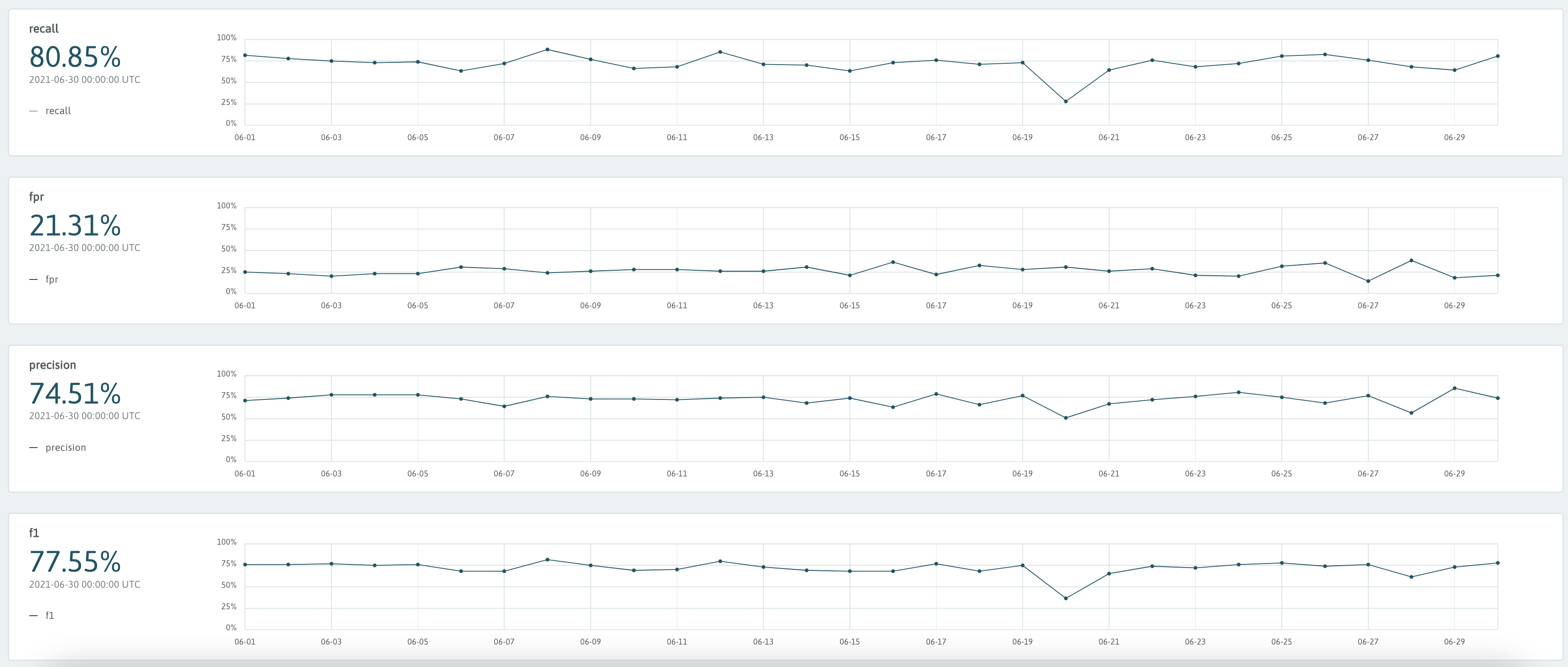# Performance Metrics

In addition to profiling datasets which feed ML models, WhyLabs can automatically track a variety of performance metrics based model predictions. In order to make this possible, the following conditions must be met.

Users must have a list of predictions and actuals, and (for classification models only) confidence scores The relevant model must have a model type declared as classification or regression.

Users can declare a model type upon creating a new model from either the Model Dashboard or the Model Management section within settings.For any models already assigned the “Unknown” model type, users can declare a new model type by editing the settings in the Model Management section within Settings.

## Regression#

In the case of regression models, the following metrics are tracked:

• Total output and input count
• Mean Squared Error
• Mean Absolute Error
• Root Mean Squared ErrorWhen logging performance metrics for Regression Models, the following code can be used.

###Use this for docs
import pandas as pd
import os
from whylogs.app import Session
from whylogs.app.writers import WhyLabsWriter
from whylogs.proto import ModelType
os.environ["WHYLABS_API_KEY"] = #whylabs api key
#initialize writer object
writer = WhyLabsWriter()
#start session
session = Session(project="demo-project", pipeline="demo-pipeline", writers=[writer])
#log performance metrics
with session.logger(tags={"datasetId": model_id}) as ylog:
ylog.log_metrics(
targets= model_targets_list,
predictions=model_predictions_list,
prediction_field="pred_vals",
target_field="target_vals",
model_type=ModelType.REGRESSION
)

Similar to other logging methods, users can optionally provide a dataset_timestamp parameter when initializing the logger in cases where backfilling is required.

See an example notebook which demonstrates the use of performance metrics for regression models.

## Classification#

In the case of classification models, the following metrics are tracked:

• Total output and input count
• Accuracy
• ROC
• Precision-Recall chart
• Confusion Matrix
• Recall
• FPR (false positive rate)
• Precision
• F1

The metrics above are supported for both binary classification and multi-class classification.The code for logging classification metrics is similar to that of regression, with addition of scores associated with each prediction. These scores are often probabilities or some other confidence measure.

with session.logger(tags={"datasetId": model_id}) as ylog:
ylog.log_metrics(
targets= model_targets_list,
predictions=model_predictions_list,
scores=model_scores,
prediction_field="pred_vals",
target_field="target_vals",
score_field="score_vals",
model_type=ModelType.CLASSIFICATION
)

See an example notebook which demonstrates the use of performance metrics for classification models.

## Performance Metrics with PySpark#

Performance metrics can also be logged with spark using the syntax below.

from whyspark import new_profiling_session
import pandas as pd
#set environment variable
%env WHYLABS_API_KEY= #api key
%env WHYLABS_ORG_ID= #org id
%env WHYLABS_MODEL_ID= #model id
#rename columns so they are correctly identified as output features
pdf = pdf.rename(columns={"delivery_prediction":"delivery_prediction (output)",
"delivery_status": "delivery_status (output)",
"delivery_confidence": "delivery_confidence (output)"})
#create spark dataframe from pandas dataframe
df = spark.createDataFrame(pdf)
#initiate session
session = new_profiling_session(df, "my-model-name")
#for classificaiton performance metrics only
classificationSession = session.withTimeColumn('order_estimated_delivery_date') \
.withClassificationModel("delivery_prediction (output)",
"delivery_status (output)",
"delivery_confidence (output)")
classificationSession.log()
#for regression performance metrics only
regressionModel = session.withTimeColumn('order_estimated_delivery_date') \
.withRegressionModel("delivery_prediction (output)",
"delivery_confidence (output)")
regressionModel.log()

See a full example of logging performance metrics with pyspark in this notebook.

Don't hesitate to reach out if you would like to log performance methods via one of our other integrations!

## Performance Comparison#

WhyLabs allows users to compare the performance of two models side by side. Users can select two models of the same type (classification or regression) in the upper left dropdowns. WhyLabs will display plots of performance metrics from each model.

This makes it a simple matter to determine the superior model when comparing multiple versions of a model.Run AI With Certainty